Thank you for visiting nature.com. You are using a browser version with limited support for CSS. To obtain the best experience, we recommend you use a more up to date browser (or turn off compatibility mode in Internet Explorer). In the meantime, to ensure continued support, we are displaying the site without styles and JavaScript.

# Coexistence of competing stage-structured populations

## Abstract

This paper analyzes the stability of a coexistence equilibrium point of a model for competition between two stage-structured populations. In this model, for each population, competition for resources may affect any one of the following population parameters: reproduction, juvenile survival, maturation rate, or adult survival. The results show that the competitive strength of a population is affected by (1) the ratio of the population parameter influenced by competition under no resource limitation (maximum compensatory capacity) over the same parameter under a resource limitation due to competition (equilibrium rate) and (2) the ratio of interspecific competition over intraspecific competition; this ratio was previously shown to depend on resource-use overlap. The former ratio, which we define as fitness, can be equalized by adjusting organisms’ life history strategies, thereby promoting coexistence. We conclude that in addition to niche differentiation among populations, the life history strategies of organisms play an important role in coexistence.

## Introduction

The dynamics of competition between two species have been studied extensively, but few of these prior studies have incorporated variation in life history strategies between competing populations. Here, we present a mechanism for the coexistence of populations with different life history strategies (species). The classic Lotka-Volterra competition model1 predicts that unless competition between two species is weak, the population that has a competitive advantage will exclude the other; this is also known as the competitive exclusion principle2,3,4. In order for two species to coexist, their interspecific competition must be weaker than their intraspecific competition (stabilizing mechanism)5. However, the amount of stabilization required for coexistence can be small if the difference in average fitness between the two species is small (equalizing mechanism)5. In other words, strong stabilization alone or weak stabilization plus strong equalization is required for species coexistence.

Many sympatric populations do not compete for a single resource, which means that stabilization maintains their coexistence. However, one of the interesting questions in ecology is how sympatric populations that do compete strongly with each other (i.e. cases in which interspecific competition is only slightly weaker than intraspecific competition) can coexist. This question may be reduced to the goal of determining how the average fitness associated with two species is equalized.

Fitness is a function of an organism’s life history parameters within its environment (e.g. ref. 6) assuming no individual heterogeneity within species beyond stage differences. There is clearly a diverse range of life history strategies among species: e.g. some mature early (precocious) while others delay maturation; some adopt semelparous reproductive strategies while others are iteroparous; and some are long-lived and others short-lived. We call this type of diversity demographic biodiversity. Although demographic biodiversity is expected to have profound effects on interactions between species, it has been largely overlooked in theoretical studies containing multiple species (exceptions include refs. 7, 8, 9, 10). A previous study10 examined a model similar to the one discussed in this paper. Their numerical analysis used two-stage Ricker models11, assumed only individuals in the same stage of two populations (i.e. between adults and between juveniles of two populations) compete with each other and showed the possible asymptotic outcomes, but did not derive the criteria for the outcomes. Here, we use two-stage Beverton-Holt models12, which can be derived by assuming competition for available resources and derive analytical criteria for existence and the stability of coexistence equilibria. This allows us to interpret the criteria in terms of biological processes. Finally, we show how demographic biodiversity can equalize the fitness of two species, thereby promoting their coexistence. To our knowledge, other previous attempts used models that are special cases of our models (e.g. refs 7, 8, 9).

We analyze a model that is comprised of two stage-structured populations of different species that experience competition for available resources at some life stage. Although the classic Lotka-Volterra competition model is formulated in continuous-time equations, we use a discrete-time model in order to include stage structured populations. The dynamics of discrete-time stage-structured population models of this type have been thoroughly studied (see ref. 13). A discrete-time version of the Lotka-Volterra competition model with the Beverton-Holt type density dependence12 has also been studied and shown to exhibit the same asymptotic dynamics as that of its continuous counterpart7. Here, we combine these two classes of models to construct a family of discrete-time, stage-structured competition models and develop a fitness measure that is useful in determining the resulting dynamics. We then relate asymptotic dynamics of the competition model to the life history strategies of the competing populations.

The rest of the paper is structured as follows. First, we describe a single-species stage-structured model and its asymptotic dynamics. Second, we develop a two-species model in which competition affects juvenile survival of one species and adult survival of the other. Third, we generalize the analysis by varying the population parameters (possibly different life history parameters for each of the two populations) affected by competition. Finally, the model results are discussed in the context of existing ecological theories of two-population competition.

## Results

### Stage-structured population model: single population

The competition model consists of two stage-structured population models coupled by a resource limitation that affects one life history rate in each population. Each stage-structured population model (Fig. 1) consists of two developmental stages: juveniles (stage 1) and adults (stage 2). In this model, for population i, juveniles survive with rate si and develop into adults with rate mi , while adults survive with rate pi and reproduce at fertility rate fi . The fertility rate is the number of offspring per adult times their rate of survival to the age of one year. The matrix Ai associated with this life history is given by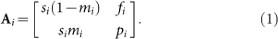This matrix, which is termed a population matrix, transitions a population vector,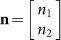, from time t to t+1 as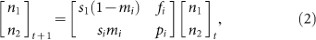where n1 and n2 are the stage densities of juveniles and adults, respectively and t denotes time. See ref. 13 for general descriptions of matrix population models.

The above stage-structured model has been used by ref. 14 to incorporate the different life history strategies of organisms. For example, when mi approaches 1, individuals tend to mature early (precocious), but when mi approaches 0, individuals delay maturation (delayed). Similarly, when pi approaches 1, individuals reproduce repeatedly (iteroparous), but when it approaches 0, individuals reproduce a small number of times or only once (semelparous). Furthermore, we can change the distribution of survivorship from birth to reproduction by changing fi and si (note that fi is the number of offspring times their survival over one time unit) and we can alter the life expectancies of individuals by changing fi , si and pi . Therefore, this two-stage model is one of the simplest stage-structured population models that can incorporate a wide range of life history strategies.

### Density dependence

In the current study, any of the four population parameters in equation (1) may experience density dependence (non-linearity) due to resource limitation. Hereafter, this density dependence is termed intraspecific competition. For a population parameter, which is generally denoted by xi , where xi is si , mi , pi , or fi , the intraspecific competition is modeled as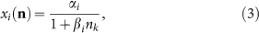where αi is the maximum of xi at a low stage density, βi is the effect of stage density (density of a given stage) on rate x and k denotes the stage (1 for juvenile and 2 for adult). We use function notation xi (n) as a reminder of density dependence throughout the paper. Whether the rate is a function of juvenile or adult density depends on which population parameter is affected by the intraspecific competition. It is assumed that intraspecific competition among juveniles affects the population parameters in the left-hand column of the population matrix (si and mi ), while competition among adults affects the parameters in the right-hand column (fi and pi ). Ecologically, this implies that juveniles and adults have separate niches.

Equation (3) is the Beverton-Holt density dependence12. We choose this form because it can be derived by assuming competition among individuals for an available resource; this is arguably one of the most common types of intraspecific competition experienced by animals and it is equivalent to the type of competition included in the classic Lotka-Volterra competition model. Furthermore, the Beverton-Holt density dependence leads to stable asymptotic dynamics. Here, we define αi as the maximum compensatory capacity of xi and βi as the intraspecific competition strength.

### Parameter constraints

The dominant eigenvalue, λD , of the population matrix (equation 1) can be solved analytically as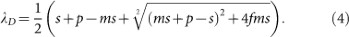Here, the subscripts for population i are omitted to avoid clutter. Any one or more of the parameters in the population matrix can experience intraspecific competition. However, due to the magnitude restrictions on the life history parameters, the dominant eigenvalue is 1 at the non-trivial equilibrium point (i.e. when the population is at a positive equilibrium). Therefore, when we set λD = 1, the four population parameters at an equilibrium must satisfy the following relationship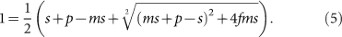This equation can be analytically solved for any of the four parameters. Figure 2 shows the relationship among three population parameters under λD = 1 when the other parameter, which is indicated by superscript (c) in the figure legend, is fixed at some constant value.

The eigenvector associated with the dominant eigenvalue gives the asymptotic distribution of the stage densities between the two stages. Therefore, at the positive equilibrium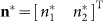, the stage 1 and stage 2 densities satisfy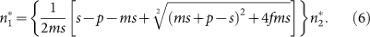Equation (6) shows that, under a given set of population parameters and the population being at a positive equilibrium point, the state of the population can be expressed in terms of a scalar density.

In addition to the above relationships at the positive equilibrium, there are also trade-offs between population parameters (e.g. ref. 6). For example, juveniles optimize their energy/resource allocation between surviving and developing into the adult stage, while adults optimize their energy/resource allocation between reproduction and survival. Although the energy/resource allocation is an active area of research (e.g. ref. 15), the trade-off function is not yet well-understood. Here, we simply suggest that it is not plausible to have either high juvenile survival and fast development or high adult survival and high fertility (Fig. 2).

### Stage-structured competition model: two populations

The stage-structured population model consists of four population parameters (si , mi , pi and fi ), any one of which may be affected by intraspecific competition. If another population uses the same resource and that particular resource limits one of the four population parameters for the second population, then there is interspecific competition. Because the parameters affected by the resource may be different for the two populations (e.g. the limitation could affect the fertility of population 1 but the maturation rate of population 2), there can be 10 different interactions between the two populations. First, we develop and analyze a model in which a potential resource limitation affects the juvenile survival of one population and the adult survival of the other population. Then, we will generalize the results for the other cases.

To build the competition model, we start with the constant, four-by-four matrix M consisting of two population matrices (equation 1) in block-diagonal entries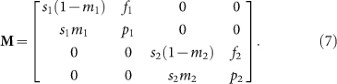Hereafter, we will call this a competition matrix. The competition matrix simultaneously transitions the stage densities of the two populations. Therefore, the first, second, third and fourth components of the vector, which we term a community vector, are the density of juveniles in the first population (n1), the density of adults in the first population (n2), the density of juveniles in the second population (n3) and the density of adults in the second population (n4), respectively.

The competition matrix is a block diagonal matrix, which has some properties that simplify the analysis. First, the eigenvalues of the matrix are given by the eigenvalues of two submatrices (i.e. eigenvalues of individual population matrices). Second, the eigenvectors have the following form: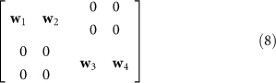where w1 and w2 are the (column) eigenvectors of the upper left submatrix and w3 and w4 are the (column) eigenvectors of the lower right submatrix. Each population matrix is a nonnegative, irreducible and primitive matrix when pi >0 or mi <1. This ensures that each submatrix has a dominant eigenvalue that is real and its magnitude is greater than that of the subdominant eigenvalue13. When pi = 0 and mi = 1, the magnitude of the two eigenvalues are the same. This is the case in which an organism is semelparous and reproduces only at age 2. Therefore, the necessary condition for the coexistence of two populations is that the dominant eigenvalues of the two submatrices are 1 at the equilibrium point. Finally, when the stage densities are at the equilibrium point, stage distribution is proportional to the eigenvectors associated with the dominant eigenvalues of the submatrices. We take advantage of these properties with the analyses of the competition matrix.

### Competition affecting the juvenile survival of one species and adult survival of the other: Existence of a non-trivial equilibrium

In this section, we assume that the juvenile survival rate of one population and the adult survival rate of the other population are limited by the same resource. Therefore, s1 and p2 are affected by both inter- and intra-specific competition, which are given as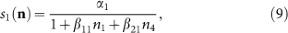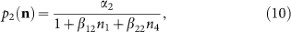where βij is the effect of population i on j and is termed interspecific competition strength. These terms are maximized at αj when the juvenile density of the first population and the adult density of the second population (two competing stages) are low. These rates, s1(n) and p2(n), are reduced as one or both of the stage densities are increased with the rate of the reduction determined by βjj and βij .

To determine the stability of an equilibrium point of a discrete-time system, a common approach is to find a linearized matrix around the equilibrium and calculate the dominant eigenvalue of the matrix. Unfortunately, although it is simple to find the linearized competition matrix, it is not trivial to obtain an analytical expression of its dominant eigenvalue unless one or both of the populations are at zero. Therefore, we analyze the model using the isocline method7. This method uses lines where λD = 1, lines of no population growth to display the existence and stability of equilibrium points. This is a discrete-time equivalent to the isocline method that is commonly used for determining the stability of the continuous-time Lotka-Volterra competition model.

At a non-trivial equilibrium, the dominant eigenvalue of each population matrix is 1. It is satisfied when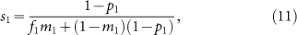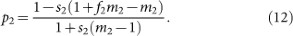These equations are obtained by solving equation (5) for si and pi . Hereafter, the juvenile and adult survival rates that satisfy equations (11) and (12) are denoted by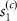and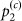, respectively. We then determine the isoclines (i.e. the lines on which the dominant eigenvalue of a population matrix is 1) using the following two equations: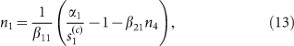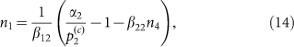which determine the isoclines for the first and second populations, respectively. These are obtained by solving equations (9) and (10) for n1 and substitutingand, respectively. Four of the possible geometries of the two isoclines are shown in Figure 3. The location where two isoclines intersect is the non-trivial equilibrium point. It should be noted that equations (13) and (14) give necessary conditions for there to be a non-trivial solution for the nonlinear, four-by-four system of equations that express an equilibrium for the density-dependent model. These conditions are not generally sufficient to conclude that such equilibrium solutions exist, but we show in Methods that when the Beverton-Holt density-dependence model is applied to any of the four basin system parameters, a non-trivial equilibrium will exist.

### Stability of the coexistence equilibrium

In Figure 3, the solid lines are the isoclines for population 1, the dashed lines are the isoclines for population 2 and the arrows show the directions of the transition by the competition matrix when the two populations are close to the stable stage distribution (i.e. asymptotic distribution). Three qualitatively different equilibrium points exist: both populations are at zero (extinction); one population is at zero and the other is positive (exclusion); and both populations are positive (coexistence). It is clear that extinction is always unstable as long as there are isoclines for the two populations. However, it should be noted that extinction can be stable when neither population has an isocline in the positive quadrant.

Figure 3 only shows isoclines as a function of two stages (n1 and n4) but not others (n2 and n3). This is because the competition terms depend only on these two stages in the model. They are the only stages that directly affect the population parameters, thus the asymptotic population growth rates. Any perturbations to the four stages, including n2 and n3, are projected as perturbations to n1 and n4; therefore, n1 and n4 can also be viewed as functions of past n2 and n3. The system will be either attracted to or repelled from the equilibrium point defined by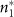and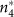. When the system is attracted to the equilibrium point, n2 and n3 will also be attracted to the values determined by the eigenvector associated with the dominant eigenvalue (which is 1) of each submatrix because of the properties of the community matrix.

The conditions for stable coexistence can be expressed in terms of population parameters (Methods) as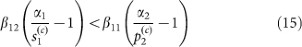and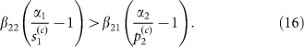Because the method for determining the stability in this study is not a common approach, we also numerically calculated the dominant eigenvalue of the linearized community matrix around the non-trivial equilibrium point. When the dominant eigenvalue is less than 1, the equilibrium point is asymptotically stable. The result was compared with the prediction from conditions (15) and (16). This procedure was repeated more than 100,000 times with randomly selected population parameters. Under all simulations, the predictions from the conditions (15) and (16) were consistent with the eigenvalue of the linearized community matrix.

The term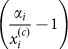in conditions (15) and (16) represents an excess production of juveniles and adults by population 1 and 2, respectively. The termis the juvenile survival rate and α 1 is the maximum survival rate when the density of individuals utilizing the resource is very small (maximum compensatory capacity) while the term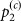is the adult survival rate and α 2 is the maximum survival rate when the density of individuals utilizing the resource is very small (maximum compensatory capacity). In general,is a function of the other population parameters that define the life history strategy (equation 5). The term αi determines how well the population performs when the resource is abundant and must satisfy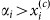. Because this term determines the average performance of individuals under the environmental conditions they experience and their life history, we define this excess production term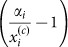as the average fitness of individuals associated with population i.

### Stability criteria

The conditions for stable coexistence are formulated similarly to the conditions found under the unstructured Lotka-Volterra competition model (e.g. refs. 5, 7). Therefore, interpretations of the Lotka-Volterra competition model can be directly applied to the results herein. This allows strong connections between the current results and existing ecological theories of two-population competitions. According to conditions (15) and (16), intraspecific competition (βii ) must be stronger than interspecific competition (βij ) in order for the two populations to coexist. It has been shown that the relative strengths of the two competition terms are determined by the resource-use overlap under the classic Lotka-Volterra competition model and this was termed a stabilizing effect5. However, this stabilization can be small if the maximum per capita growth rate of the population relative to the rate at which this growth rate declines with the density, which was also termed fitness in ref 5, is similar between two populations and this mechanism was termed an equalizing effect5. Similarly to previous results, our results suggest that when the excess production terms, which we define as the fitness terms in our model, between the two populations are close to each other, only a small level of stabilizing effect is needed for the coexistence of the two populations. On the other hand, if the fitness associated with population 1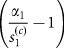in our model becomes much greater than that of population 2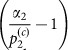, the direction of inequality reverses (Fig. 3b) and population 1 always excludes population 2. Similarly, if the fitness associated with population 2 becomes much greater than that of population 1, the direction of inequality reverses (Fig. 3c) and population 2 always excludes population 1. Finally, if interspecific competition (βij ) becomes stronger than intraspecific competition (βii ) for both populations, the directions may switch for both inequalities (Fig. 3d). If this occurs, the two exclusion equilibria are both stable and the final state depends on the initial population densities (alternative steady states).

The new criteria developed in this analysis include the fitness terms that are also explicit functions of life history parameters allowing us to interpret the criteria in terms of the population parameters. The fitness increases with the maximum compensatory capacity of the juvenile or adult survival rate and decreases with the equilibrium rate. In other words, organisms that have a high potential for survival under low resource competition but do not depend on this high survival rate at an equilibrium (i.e. at the carrying capacity of the environment) are competitively superior. Under the assumption that the maximum compensatory capacities associated with juvenile survival of population1 and adult survival of population 2 are close to 1 (or similar between the two), the fitness increases when the equilibrium rate decreases.

The equilibrium juvenile survival rate () is maintained at a low level by two general life history strategies (Fig. 2a): (1) a high adult survival rate (pi ) or (2) when the adult survival rate is low, a high fertility rate (fi ) or a reduced fertility rate that is compensated for by an increased maturation rate (mi ). In Figure 2a, when the adult survival rate is high, the maturation rate (contour line) is low and fertility (vertical axis) can also be low. Any increase in either or both of these values will allow further reduction in the equilibrium juvenile survival rate. Therefore, high adult survival is one sufficient life history strategy. On the other hand, when the adult survival rate is low, there must be a sufficiently high fertility rate or maturation rate in order to maintain a low juvenile survival rate at the coexistence equilibrium. In other words, strategies to maintain a large number of individuals in an adult stage or to increase the number of individuals maturing are more advantageous.

On the other hand, the adult survival rate at the coexistence equilibrium (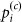) is maintained at a low level by two general life history strategies: (1) high fertility rate or (2) high juvenile survival rate (Fig 2d). Figure 2d shows that when the fertility rate is high, the juvenile survival and maturation rate can be low. Any increase in either or both of these values will allow further reduction in the equilibrium adult survival rate. Similarly, when the juvenile survival rate is high, both maturation and fertility rate can be low although there is some trade-off between the two. In other words, a strategy to accumulate individuals in the juvenile stage is more advantageous.

### Stability of the coexistence equilibrium in the general two species competition model

The results in the previous section, in which the juvenile survival rate of one population and adult survival rate of the other exhibit density dependence, can be generalized to other cases, including those in which competition affects different population parameters. Here, rates s1 and p2 are simply replaced with si , mi , pi , or fi and isoclines can be plotted against stages that are directly affected by the competition. As long as the directions of the arrows on the isocline figures remain the same, the results in the previous section hold. This is the case when the dominant eigenvalue of the population matrix (equation 1) evaluated at the equilibrium is an increasing function of the population parameters. This condition is satisfied for si , pi and fi because these parameters are always positively associated with an entry in a population matrix. Furthermore, the derivative of λD with respect to mi at λD = 1 is always positive (Methods).

The effects of resource limitation in each of the four population parameters are summarized in Table 1. When the fertility rate (fi ) is affected by a resource limitation, high adult survival can compensate (Fig. 2b). Alternatively, high juvenile survival with some tradeoff between adult survival and maturation rate can also maintain high fitness. The fertility rate is unique in that its maximum compensatory capacity αi can be higher than 1. However, this does not mean that organisms that maximize the number of offspring (fecundity) are always at a competitive advantage because the fertility term is the product of fecundity and offspring survival until age 1. Some organisms may produce a large number of offspring with relatively low survival, while others may produce fewer offspring with relatively high survival16. The maximum compensatory capacity can be increased effectively by optimizing the trade-off between the two parameters rather than by simply increasing fecundity.

If the maturation rate (mi ) is affected by a resource limitation, fitness is high when individuals can mature early without a resource limitation (high maximum compensatory capacity) but do not need to mature early when the stage density is saturated at an equilibrium (low equilibrium rate). A low equilibrium maturation rate can be achieved by a high juvenile survival rate (Fig. 2c), but as the juvenile survival rate decreases, the trade-off between adult survival and fertility becomes stronger. When the juvenile survival and maturation rates are very low, only a high adult survival rate can maintain a stable coexistence equilibrium.

## Discussion

Here, we extended the classic Lotka-Volterra competition model to include stage structures within each of two competing populations and show that organisms can change competitive strength by adjusting their life history parameters. In particular, we find that equilibrium rates are a function of the life history parameters that are not directly affected by resource limitation. By adjusting these parameters, organisms can maximize their fitness under various behavioral, morphological, physiological and environmental constraints. This, in turn, promotes the coexistence of the two populations. The premise in the last argument is that maximization of fitness in the presence of constraints will tend to equalize fitness among competing populations under resource constraints. Although this makes intuitive sense, further research into life history trade-offs under various constraints will be needed to firmly link fitness maximization to equalization. For example, investigating how organisms increase the fertility rate by optimizing the number and quality of their offspring and determining a range of feasible fertility rate for given organisms may be interesting topics.

The coexistence of two species is typically attributed to niche differentiation (stabilization)5. However, although our model does require some stabilization in order for the two species to coexist, this stabilization can be small if the two species have similar levels of fitness (equalization) and their differences in life history can promote equalization. Equal fitness between two species is equivalent to there being neutrality between them17. Therefore, we suggest that the question of whether stabilization or equalization is more important is equivalent to asking which is more important, niche differentiation18 or neutrality17. Another important contribution of the current study is our finding that demographic biodiversity can promote equalization, which contrasts with the general notion that demographic biodiversity reduces neutrality.

The model examined in this study assumes that the population parameters that are unaffected by competition are constant on an ecological time-scale. In reality, however, density dependence affects multiple population parameters and changes the rates. For example, a competition that strongly affects juvenile survival will reduce its density, which will lead to subsequent reduction in adult density. However, reduced adult density can increase adult survival and/or fertility if these parameters are affected by density dependence. In other words, organisms are expected to have plasticity in population parameters. This may in turn help equalization of the fitness between two populations, thereby promoting their coexistence.

The model also assumes that there are only two stages in a population. However, competition or other density dependence may affect only a portion of individuals in one of the stages (e.g. younger or older individuals within a stage). Then, further division of stages may be more appropriate. If so, competition for resources may potentially lead to more complex life history strategies.

Our results emphasize the importance of demographic biodiversity and have critical implications in conservation biology. Many theoretical ecologists have sought to examine systems containing more than two interacting populations19,20,21,22,23. Our results suggest the importance of including organisms with various life history strategies in the system with competition affecting different life history parameters. We propose that, in addition to resource partitioning (e.g. ref 23), demographic biodiversity plays an important role in determining the successful coexistence of multiple populations.

## Methods

### Existence of non-trivial equilibrium point

When the Beverton-Holt density dependence reflecting dependence on one stage, juveniles (n1 and n3) or adults (n2 and n4), is applied to one life history parameter (s, m, p, or f) and the beta vectors for the influence of each population (i.e.and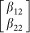, also see equation (3)) are linearly independent, a non-trivial equilibrium will exist. To demonstrate this, we use previously proven conditions of the model to construct a non-trivial equilibrium vector n* in the case where juvenile survival of population 1 (s1) and adult survival of population 2 (p2) exhibits the Beverton-Holt density dependence. The other cases follow similarly.

Since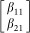and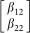are linearly independent, we may define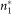and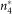as follows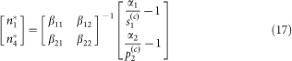where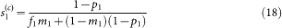and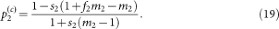Note equations (18) and (19) are identical to equations (10) and (11) from the text.

We definewhereare population matrices of the form found in equation (1) with parameters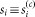, mi , pi and fi for i = 1 and si , mi ,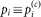and fi for i = 2.

Set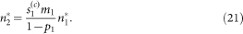Then,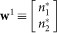satisfies the eigenequation w1 = A1w1.

Similarly, set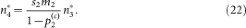Then, w2 = A2w2 where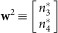.

Revisiting M, we see that by rearranging (17),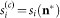and M = M(n*). Combining the definition of n* with (20)–(22), we have n* = M(n*)n*, which gives us a non-trivial equilibrium n*.

### Stability of the coexistence equilibrium point

The existence and stability of equilibria under the stage-structured competition model (equation 7) depends on the relative locations of the intercepts that the two isoclines form with the two axes (Fig. 3). The intercepts of the population 1 and 2 isoclines with the vertical axes, y1 and y2, respectively, are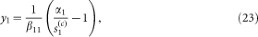and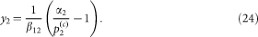Similarly, the intercepts of the population 1 and 2 isoclines with the horizontal axes, z1 and z2, respectively, are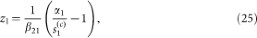and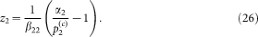In order for the coexistence equilibrium to exist, the two isoclines must intersect with each other. The conditions necessary for the coexistence equilibrium are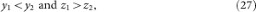or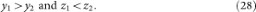Condition (27) corresponds to Figure 3a and Inequalities 14 and 15 and the coexistence equilibrium is stable. In contrast, condition (28) corresponds to Figure 3d and the coexistence equilibrium is unstable. Under condition (28), both exclusion equilibria are stable and the system will be attracted to one or the other depending on the initial condition, creating alternative stable states. Figure 3b corresponds to the condition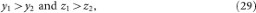where population 1 always excludes population 2 (exclusion). Similarly, Figure 3c corresponds to the condition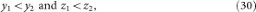where population 2 always excludes population 1 (exclusion). Table 2 lists the conditions for all possible geometries of the two isoclines when both of them exist and their associated asymptotic dynamics.

### The slope of population growth rate with respect to maturation rate

Here, we show that the derivative of the dominant eigenvalue λD with respect to m is positive. First, by taking the derivative of λD (equation 4) with respect to m, we getBy rearranging equation (4), we obtain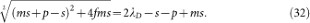Then, by substituting equation (32) into equation (31) and rearranging the equation, we generate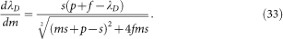The left hand side is positive when λD < p + f. This implies that the per-capita contribution of adults is greater than the asymptotic population growth rate. The contributing stage includes both juvenile and adult stages. When λD = 1, the above inequality is satisfied because adults must at least replace themselves in number as some of the replacements are juveniles that do not reproduce until they survive to reach the adult stage. Therefore, λD is an increasing function of mi around the isoclines.

## References

• Lotka, A. J. Analytical note on certain rhythmic relations in organic systems. Proc. Nat. Acad. Sci. USA 6, 410–415 (1920).

• Gause, G. F. Experimental studies on the struggle for existence: I. Mixed population of two species of yeast. J. Exp. Biol. 9, 389–402 (1932).

• Hardin, G. Competitive exclusion principle. Science 131, 1292–1297 (1960).

• Hutchinson, G. E. The paradox of the plankton. Am. Nat. 95, 137–145 (1961).

• Chesson, P. Mechanisms of maintenance of species diversity. Annu. Rev. Ecol. Syst. 31, 343–366 (2000).

• Stearns, S. C. The evolution of Life Histories. Oxford University Press (1992).

• Hassell, M. P. & Comins, H. N. Discrete-time models for 2-species competition. Theor. Popul. Biol. 9, 202–221 (1976).

• Mougi, A. & Nishimura, K. Coexistence of competitive species with a stage-structured life cycle. Ecol. Res. 20, 581–589 (2005).

• Cushing, J., Henson, S. M. & Roeger, L. Coexisting of competing juvenile-adult structured populations. Journal of Biological Dynamics 1, 201–231 (2007).

• Moll, J. D. & Brown, J. S. Competition and coexistence with multiple life-history stages. Am. Nat. 171, 839–843 (2008).

• Ricker, W. E. Stock and recruitment. J. Fish. Res. Board. Can. 11, 559–623 (1954).

• Beverton, R. J. H. & Holt, S. J. On the Dynamics of Exploited Fish Populations. Ministry of Agriculture, Fisheries and Food, London, republished by Chapman & Hall in 1993 (1957).

• Caswell, H. Matrix Population Models: Construction, Analysis and Interpretation. Sinauer Associates, Inc., Sunderland, (2001).

• Neubert, M. G. & Caswell, H. Density-dependent vital rates and their population dynamic consequences. J. Math. Biol. 41, 103–121 (2000).

• Nisbet, R. M., Muller, E. B., Lika, K. & Kooijman, S. A. L. M. From molecules to ecosystems through dynamic energy budget models. J. Anim. Ecol. 69, 913–926 (2000).

• Winemiller, K. O. & Rose, K. A. Why do most fish produce so many tiny offspring. Am. Nat. 142, 585–603 (1993).

• Hubbell, S. P. A unified theory of biogeography and relative species abundance and its application to tropical rain forests and coral reefs. Coral Reefs. 16, S9–S21 (1997).

• Hutchinson, G. E. Concluding remarks. Cold Spring Harbor Symposia on Quantitative Biology 22, 415–427 (1957).

• Cohen, J. E. et al. Improving food webs. Ecology. 74, 252–258 (1993).

• Yodzis, P. Diffuse effects in food webs. Ecology. 81, 261–266 (2000).

• Loreau, M. et al.,Ecology – Biodiversity and ecosystem functioning: Current knowledge and future challenges. Science. 294, 804–808 (2001).

• Cohen, J. E., Schittler, D. N., Raffaelli, D. G. & Reuman, D. C. Food webs are more than the sum of their tritrophic parts. Proc. Nat. Acad. Sci. USA. 106, 22335–22340 (2009).

• Rudolf, V. H. W. & Lafferty, K. D. Stage structure alters how complexity affects stability of ecological networks. Ecol. Lett. 14, 75–79 (2011).

## Acknowledgements

We thank editor and anonymous reviewers for providing comments. MF and JW were supported in part by Award No. KUS-C1-016-04, made by King Abdullah University of Science and Technology (KAUST). GP, MB and JW were supported in part by the NSF REU program DMS-0850470. GP and SD were supported by NSF DMS-0811370.

## Author information

Authors

### Contributions

All authors worked on the analyses and reviewed the manuscript. MF and GP wrote the main manuscript text. MF Prepared figures.

## Ethics declarations

### Competing interests

The authors declare no competing financial interests.

## Rights and permissions

Reprints and Permissions

Fujiwara, M., Pfeiffer, G., Boggess, M. et al. Coexistence of competing stage-structured populations. Sci Rep 1, 107 (2011). https://doi.org/10.1038/srep00107

• Accepted:

• Published:

• DOI: https://doi.org/10.1038/srep00107

• ### Robust permanence for ecological equations with internal and external feedbacks

• Swati Patel
• Sebastian J. Schreiber

Journal of Mathematical Biology (2018)